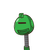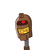# The quadratic equation $$4 {x }^{2} + 2 \sqrt{5}x + 1equal to 0$$has 1. two distinct roots 2. two

$$4 {x }^{2} + 2 \sqrt{5}x + 1equal to 0$$
has
1. two distinct roots
2. two equal real roots
3. no real roots
4. more than 2 real roots

### 2 thoughts on “The quadratic equation <br />$$4 {x }^{2} + 2 \sqrt{5}x + 1equal to 0$$<br />has <br />1. two distinct roots <br />2. two”

1.1. Two distinct roots.

Step-by-step explanation:

Since , highest power of the equation is 2 so the equation will have only 2 roots .

So 4th option will not be the answer.

so now,according to Discriminant method

D = b^2 – 4ac

D = { [2(sqrt 5)]^(2) }- 4×4×1

D= (4×5) – 16

D= 20 – 16

D= 4

Therefore,

D > 0

Hence, the given equation has real and unequal roots.

So the 2nd and 3rd options are wrong, too

So now only the option remaining is the first.

Hence option 1st is the right answer.

## I have tried to explain in the easiest way I can .

2.1. two distinct roots

Step-by-step explanation:

$$4 {x}^{2} + 2 \sqrt{5} + 1 = 0$$

D= (2√5)²- 4(4)(1)

= 20-16

=4

So this equation has two distinct roots.

## Hope this will help u...

Plz mark the answer as brainliest nd...